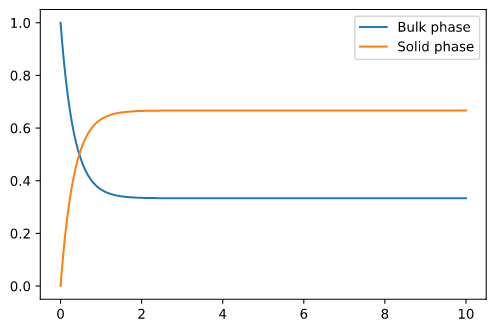# Issue with rapid equilibrium

During the workshop, I realized something odd about using the rapid equilibrium in adsorption models.

If I set the following initial conditions in a CSTR (without any in- or outgoing flow)

``````linear_model.root.input.model.unit_000.init_c = 
linear_model.root.input.model.unit_000.init_q = 
``````

and set `is_kinetic` to True

``````## Adsorption
linear_model.root.input.model.unit_000.nbound = 

``````

I get a graph that looks as expected:However, if i set `is_kinetic = False`, it seems to ignore the initial conditions. Mass is created from no where (expected would be 0.66 in solid phase and 0.33 in bulk phase).I assume this might be caused by the consistent initialization.

This sounds like a consistent initialization problem, indeed.
@j.schmoelder can you tell me the porosity to reproduce this problem?

For this example, I set the porosity to 0.5

Issue identified; Discussion continues on Github: